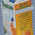## Tuesday, July 15, 2008

### An interesting summation- new?

I recently came across the following sum in my research:

&sumi (-1)i (k choose i)(a+i)k (Where the sum goes from i=0 to i=k.)

I had reason to believe that this summed to (-1)kk! (which is independent of a). A mathmatician from Univ of MD, Brian Hunt, proved it for me and I wrote it up here.
1. Is this result already known? I suspect yes but was unable to find it.
2. I was unable to find a table of known sums on the web. Does anyone know of one?
3. The techniques of the book A=B do not seem to apply. Does some modification of them suffice?
4. Is there a combinatorial proof where you show that both sides solve the same problem? Is there a more elegant proof?

1.In your proof, did you figure f '_j(x) = f_j(x)? I think there's a " ' " somewhere missing. Haven't seen this one before. I second the vote for a "sums" wiki

2.YES that should be
f_{j+1} = xf_j'(x).

I have fixed it.

As for SUMS wiki: might
be a good idea, but first
check that the sum is not
in the A=B class, since those
can ALL be done.

Bill G.

3.Hi Bill,

A more general version of this sum is in Concrete Mathematics (Knuth et al., Concrete Mathematics, 2nd Edition, page 190):

Identity 5.42:

\sum (n choose k) * (-1)^k * (a_0 + a_1*k + a_2 + ... + a_n*k^n) = (-1)^n * n! * a_n

where a_0,...,a_n are arbitrary coefficients.

4.You can poke around at the online encyclopedia of integer sequences for related results.

http://www.research.att.com/~njas/sequences/

You can look up sequences by entering the first few numbers in the sequence.

Neal

5.It's hard to come up with any sort of identity that's not already been published in Concrete Mathematics (albeit probably in a more general form)!

6.This turns out to be a familiar fact in disguise, from the calculus of finite differences: the k-th difference of the k-th power function is k! (no matter which point a you evaluate it at). So it's definitely well known, although I don't know a formal name for it. It all comes down to the alternating binomial coefficient expansion of the k-th difference of a function.

One can also give a combinatorial proof (when a is a positive integer, that is). Here's a sketch. Suppose we want to choose an ordered k-tuple of elements of {1,2,...,k+a} such that each element of {1,2,...,k} is used at least once. Of course, there are k! ways to do this. Suppose we try to calculate this by inclusion-exclusion. There are (a+k)^k sequences with no constraint. For each element of {1,2,...,k}, there are (a+k-1)^k that don't use it, so we'd better subtract off (k choose 1)*(a+k-1)^k. However, now we've oversubtracted, so we'd better add back in (k choose 2)*(a+k-2)^k to compensate. Carrying this out until the end gives the desired identity.

However, I think the calculus of finite differences interpretation is the simplest and clearest.

7.As previously mentioned see Concrete Math (my favorite book). Kind of reminds me of the sieve formula for poly-Bernoulli numbers. http://www.integers-ejcnt.org/vol8.html (A2, Page 4, equation 1)